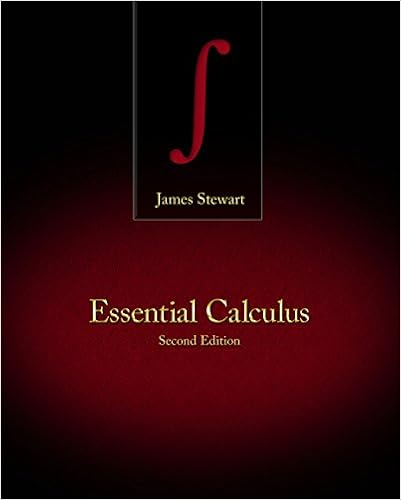# Ex 2: Find any numbers that would be excluded from the...

• Notes
• mtiwari
• 7

This preview shows page 1 - 3 out of 7 pages.

##### We have textbook solutions for you!
The document you are viewing contains questions related to this textbook.The document you are viewing contains questions related to this textbook.
Chapter 5 / Exercise 1
Essential Calculus
StewartExpert Verified
1 MA 152 Lesson 9 Section P.6 I Rational Expressions If x and y are real numbers ( 0 y ), the quotient y x is called a fraction or rational expression. Ex 1: Evaluate the following rational expression if x = 12 2 4 3 1 2 ( 5) x x x x - + - Remember, a fraction (or rational expression) cannot have a zero denominator. (Division by zero is undefined.) If a denominator has a variable, that variable cannot be any value that makes the denominator equal zero. The set of numbers for which a rational expression would be defined is called the domain. Any value of the variable which makes a denominator equal zero must be excluded from the domain. For example, the domain of the following rational expression would exclude 2 and 4 - , since those numbers make a factor of the denominator equal zero. 2 2 1 2 1 2 8 ( 4)( 2) 4 0 2 0 4 2 x x x x x x x x x x + + = - - - + - + ≠ - The domain of this expression excludes 2 and 4 - . Ex 2: Find any numbers that would be excluded from the domain of each rational expression. 2 3 ) 3 a x x +
##### We have textbook solutions for you!
The document you are viewing contains questions related to this textbook.The document you are viewing contains questions related to this textbook.
Chapter 5 / Exercise 1
Essential Calculus
StewartExpert Verified
2 2 2 2 ) 5 6 5 2 ) 4 1 x b x x x c x + + + + - II Simplifying Rational Expression 1. Factor the numerator and denominator completely. 2. Divide both numerator and denominator by any common factors. *Note: Determine what values would be excluded from the domain prior to dividing out any common factors.
•••